Скачать презентацию Risk Analysis for Portfolios Analytica Users Group Modeling

8cfb70f091092ceb21c74366cb52d59e.ppt

• Количество слайдов: 24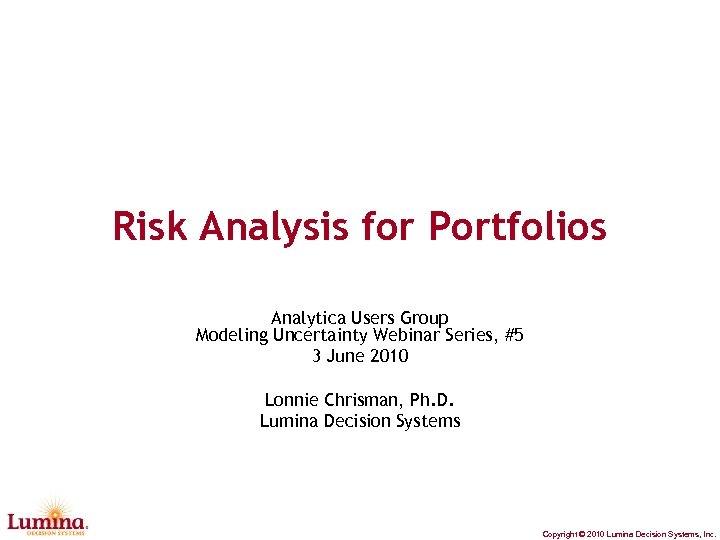Risk Analysis for Portfolios Analytica Users Group Modeling Uncertainty Webinar Series, #5 3 June 2010 Lonnie Chrisman, Ph. D. Lumina Decision Systems Copyright © 2010 Lumina Decision Systems, Inc.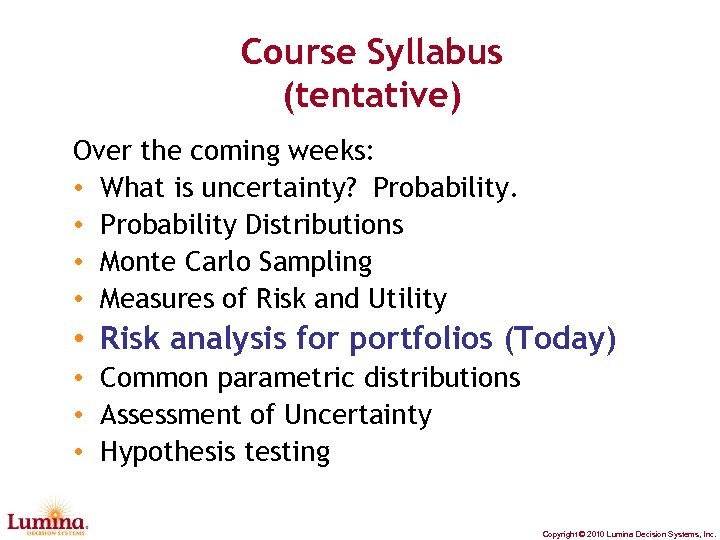Course Syllabus (tentative) Over the coming weeks: • What is uncertainty? Probability. • Probability Distributions • Monte Carlo Sampling • Measures of Risk and Utility • Risk analysis for portfolios (Today) • Common parametric distributions • Assessment of Uncertainty • Hypothesis testing Copyright © 2010 Lumina Decision Systems, Inc.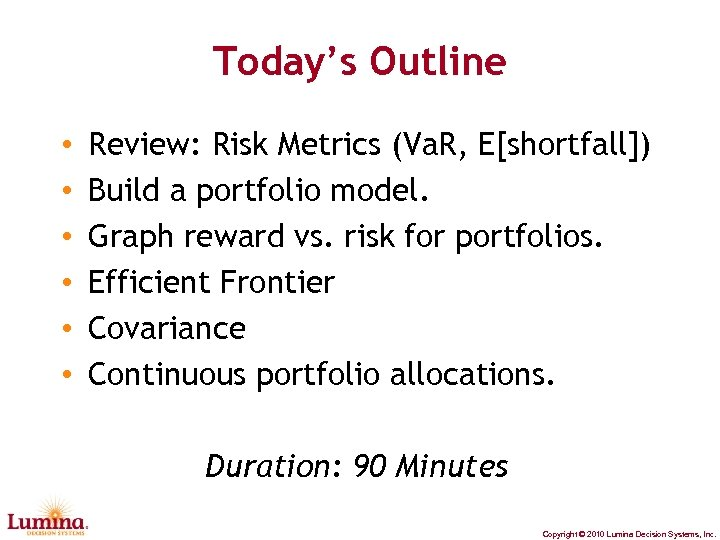Today’s Outline • • • Review: Risk Metrics (Va. R, E[shortfall]) Build a portfolio model. Graph reward vs. risk for portfolios. Efficient Frontier Covariance Continuous portfolio allocations. Duration: 90 Minutes Copyright © 2010 Lumina Decision Systems, Inc.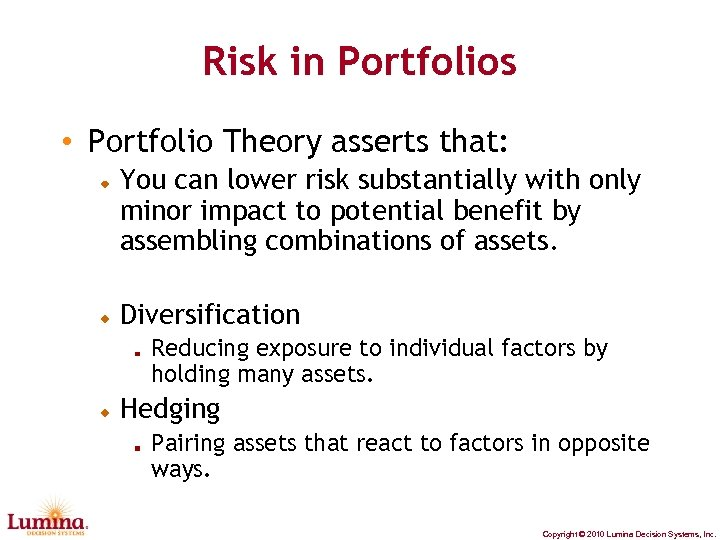Risk in Portfolios • Portfolio Theory asserts that: You can lower risk substantially with only minor impact to potential benefit by assembling combinations of assets. Diversification Reducing exposure to individual factors by holding many assets. Hedging Pairing assets that react to factors in opposite ways. Copyright © 2010 Lumina Decision Systems, Inc.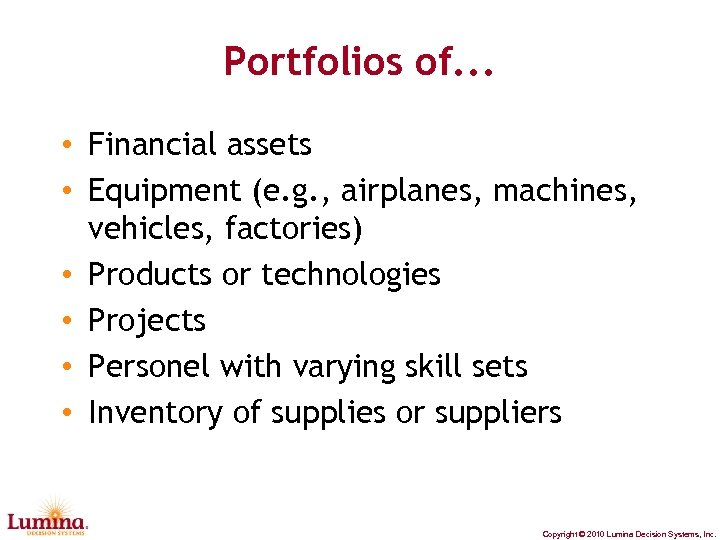Portfolios of. . . • Financial assets • Equipment (e. g. , airplanes, machines, vehicles, factories) • Products or technologies • Projects • Personel with varying skill sets • Inventory of supplies or suppliers Copyright © 2010 Lumina Decision Systems, Inc.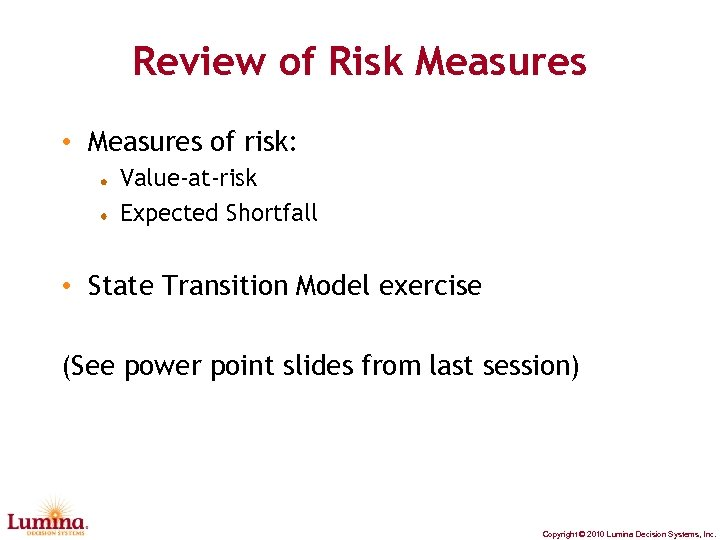Review of Risk Measures • Measures of risk: Value-at-risk Expected Shortfall • State Transition Model exercise (See power point slides from last session) Copyright © 2010 Lumina Decision Systems, Inc.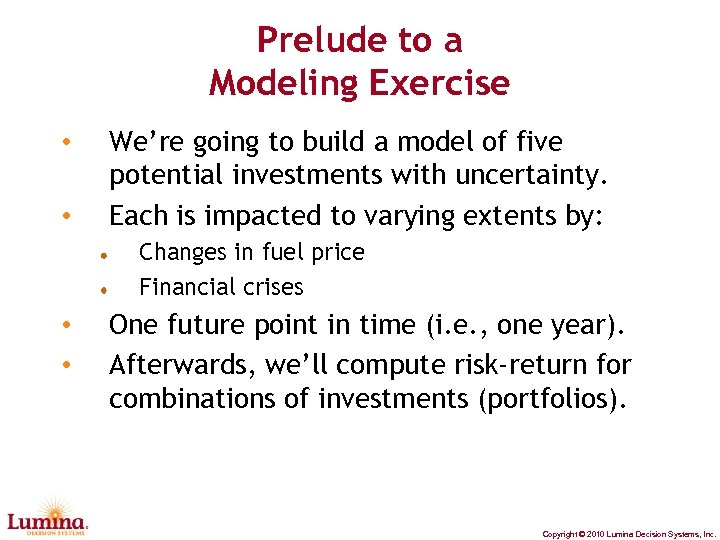Prelude to a Modeling Exercise • • We’re going to build a model of five potential investments with uncertainty. Each is impacted to varying extents by: Changes in fuel price Financial crises • • One future point in time (i. e. , one year). Afterwards, we’ll compute risk-return for combinations of investments (portfolios). Copyright © 2010 Lumina Decision Systems, Inc.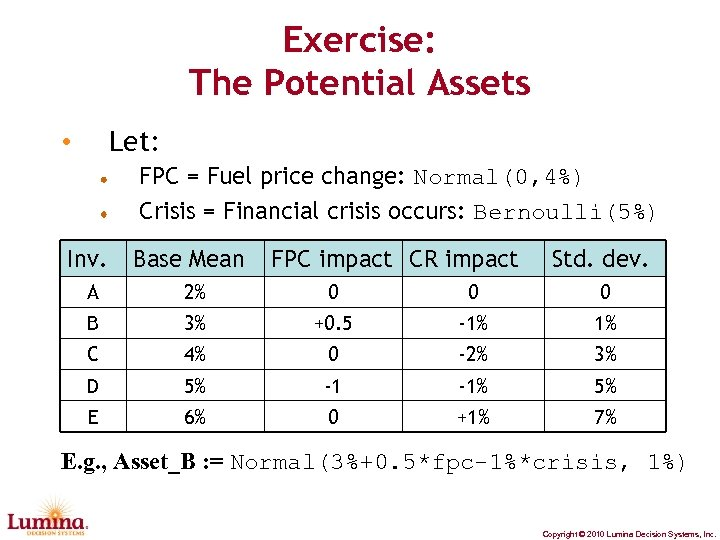Exercise: The Potential Assets Let: • FPC = Fuel price change: Normal(0, 4%) Crisis = Financial crisis occurs: Bernoulli(5%) Inv. Base Mean FPC impact CR impact Std. dev. A 2% 0 0 0 B 3% +0. 5 -1% 1% C 4% 0 -2% 3% D 5% -1 -1% 5% E 6% 0 +1% 7% E. g. , Asset_B : = Normal(3%+0. 5*fpc-1%*crisis, 1%) Copyright © 2010 Lumina Decision Systems, Inc.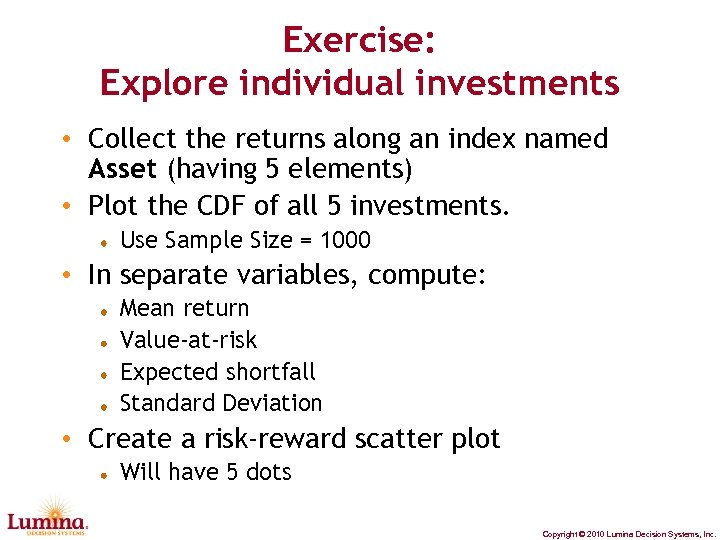Exercise: Explore individual investments • Collect the returns along an index named Asset (having 5 elements) • Plot the CDF of all 5 investments. Use Sample Size = 1000 • In separate variables, compute: Mean return Value-at-risk Expected shortfall Standard Deviation • Create a risk-reward scatter plot Will have 5 dots Copyright © 2010 Lumina Decision Systems, Inc.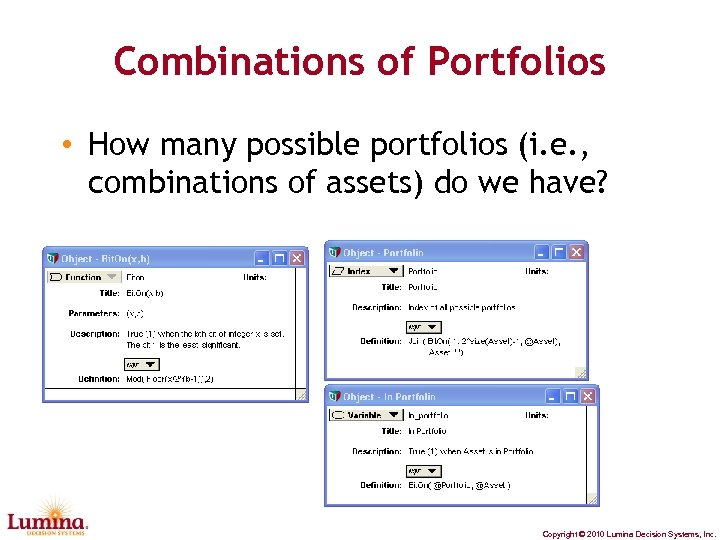Combinations of Portfolios • How many possible portfolios (i. e. , combinations of assets) do we have? Copyright © 2010 Lumina Decision Systems, Inc.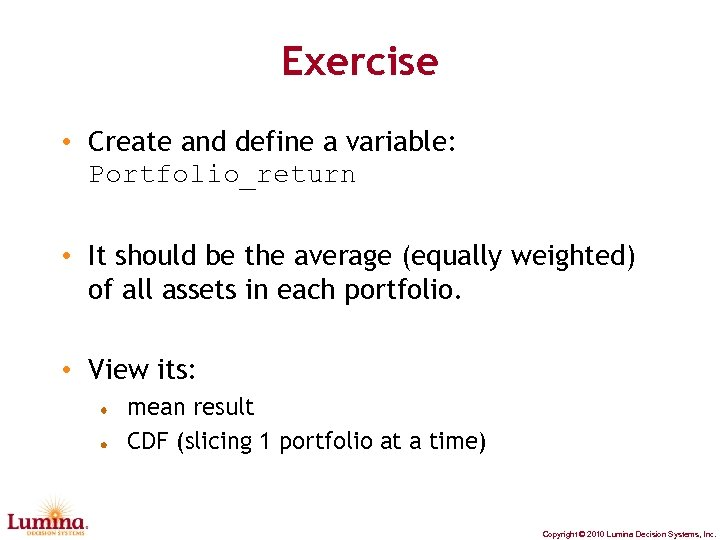Exercise • Create and define a variable: Portfolio_return • It should be the average (equally weighted) of all assets in each portfolio. • View its: mean result CDF (slicing 1 portfolio at a time) Copyright © 2010 Lumina Decision Systems, Inc.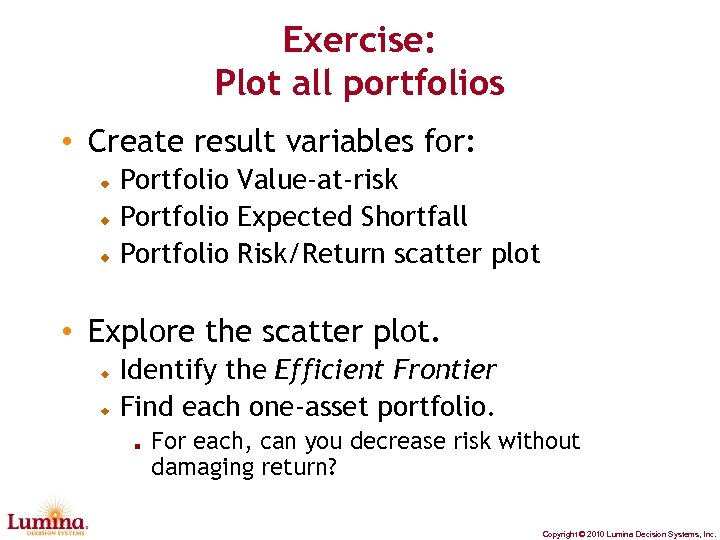Exercise: Plot all portfolios • Create result variables for: Portfolio Value-at-risk Portfolio Expected Shortfall Portfolio Risk/Return scatter plot • Explore the scatter plot. Identify the Efficient Frontier Find each one-asset portfolio. For each, can you decrease risk without damaging return? Copyright © 2010 Lumina Decision Systems, Inc.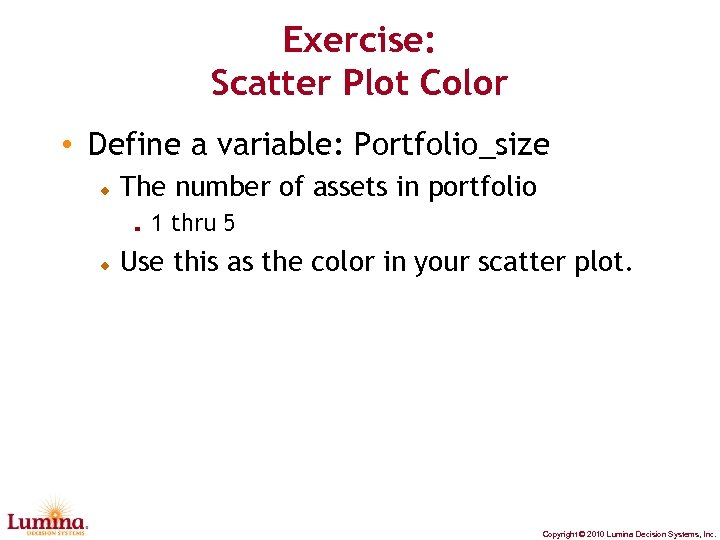Exercise: Scatter Plot Color • Define a variable: Portfolio_size The number of assets in portfolio 1 thru 5 Use this as the color in your scatter plot. Copyright © 2010 Lumina Decision Systems, Inc.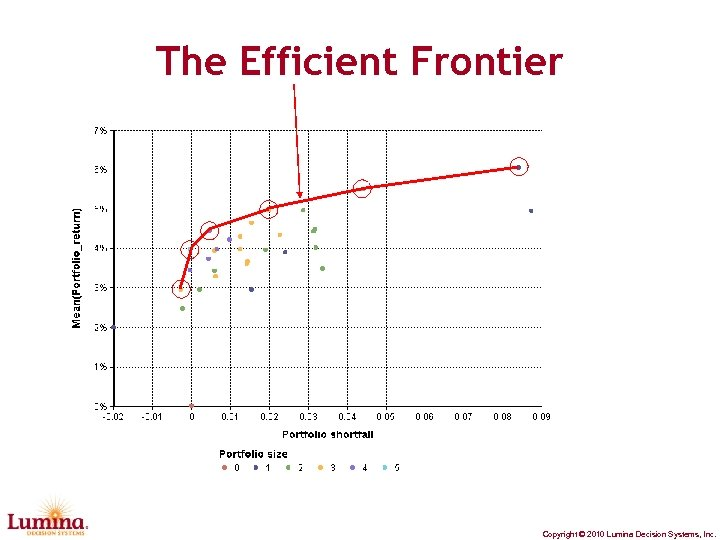The Efficient Frontier Copyright © 2010 Lumina Decision Systems, Inc.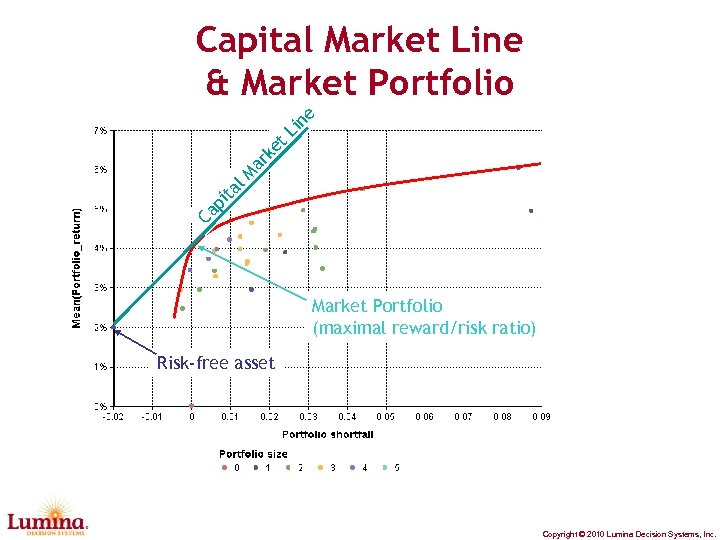Ca p ita l. M ar ke t. L in e Capital Market Line & Market Portfolio (maximal reward/risk ratio) Risk-free asset Copyright © 2010 Lumina Decision Systems, Inc.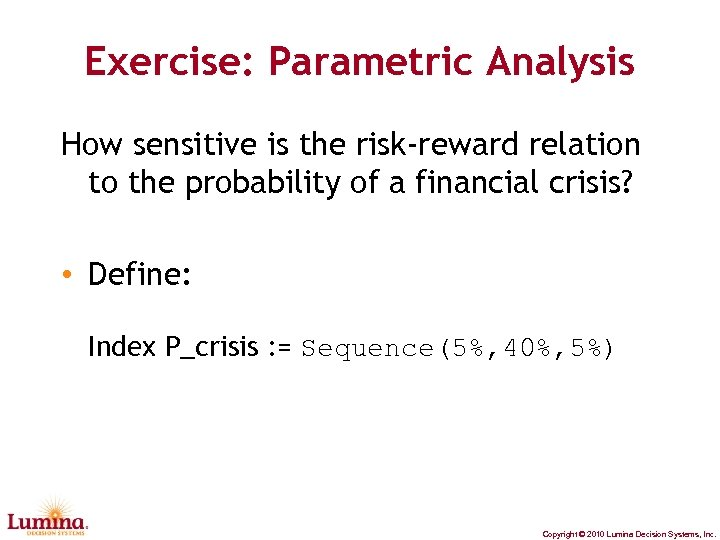Exercise: Parametric Analysis How sensitive is the risk-reward relation to the probability of a financial crisis? • Define: Index P_crisis : = Sequence(5%, 40%, 5%) Copyright © 2010 Lumina Decision Systems, Inc.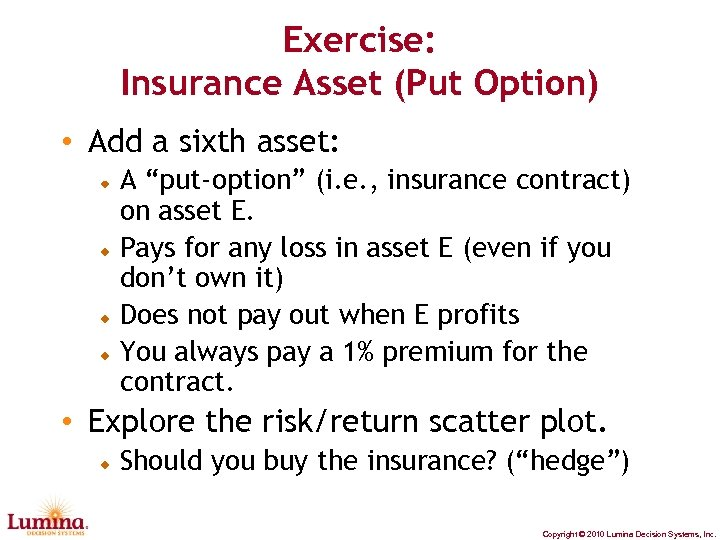Exercise: Insurance Asset (Put Option) • Add a sixth asset: A “put-option” (i. e. , insurance contract) on asset E. Pays for any loss in asset E (even if you don’t own it) Does not pay out when E profits You always pay a 1% premium for the contract. • Explore the risk/return scatter plot. Should you buy the insurance? (“hedge”) Copyright © 2010 Lumina Decision Systems, Inc.Comparison to Markowitz Portfolio Theory • Harry Markowitz (1952) Statitionary Gaussian distributions Mean & covariance matrix Reward=Mean Risk=Standard Devation Continuous allocations • Today’s presentation Structured models, arbitary distributions Reward, Risk = Any measure. Binary (yes, no) allocations. Copyright © 2010 Lumina Decision Systems, Inc.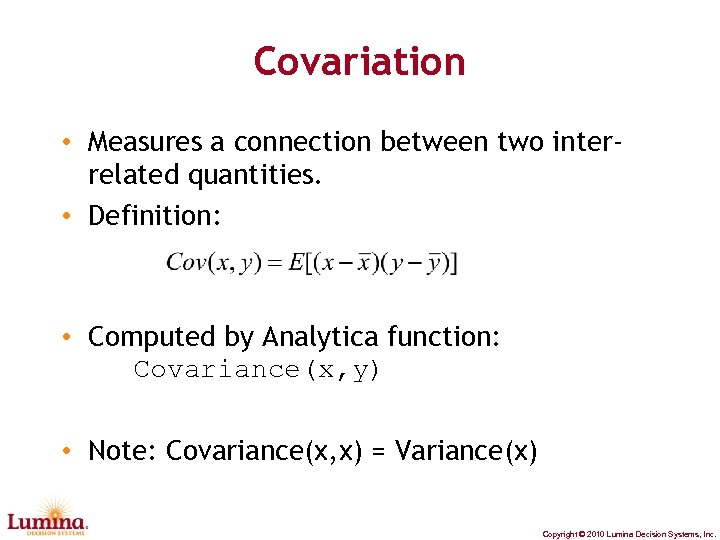Covariation • Measures a connection between two interrelated quantities. • Definition: • Computed by Analytica function: Covariance(x, y) • Note: Covariance(x, x) = Variance(x) Copyright © 2010 Lumina Decision Systems, Inc.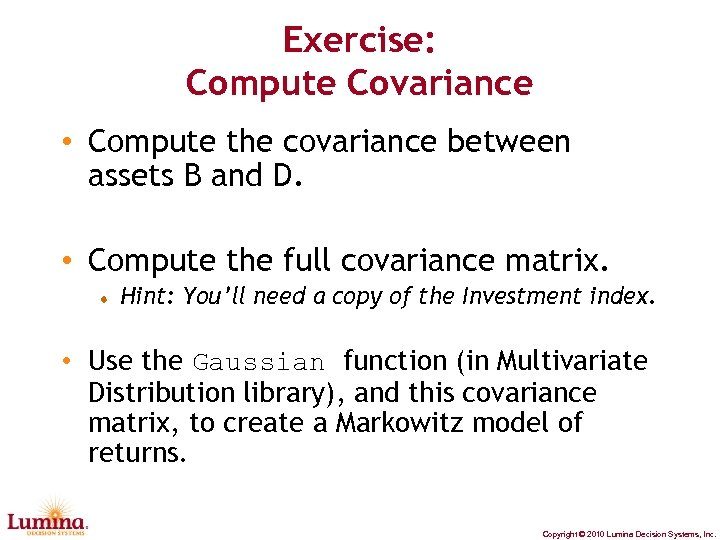Exercise: Compute Covariance • Compute the covariance between assets B and D. • Compute the full covariance matrix. Hint: You’ll need a copy of the Investment index. • Use the Gaussian function (in Multivariate Distribution library), and this covariance matrix, to create a Markowitz model of returns. Copyright © 2010 Lumina Decision Systems, Inc.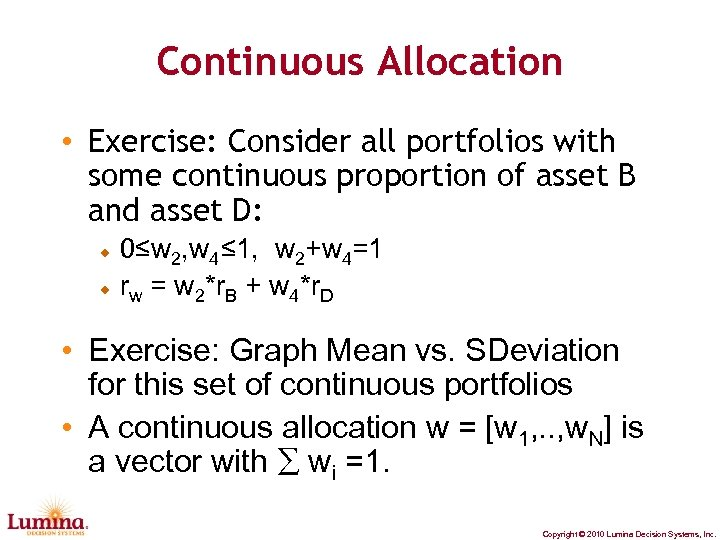Continuous Allocation • Exercise: Consider all portfolios with some continuous proportion of asset B and asset D: 0≤w 2, w 4≤ 1, w 2+w 4=1 rw = w 2*r. B + w 4*r. D • Exercise: Graph Mean vs. SDeviation for this set of continuous portfolios • A continuous allocation w = [w 1, . . , w. N] is a vector with ∑ wi =1. Copyright © 2010 Lumina Decision Systems, Inc.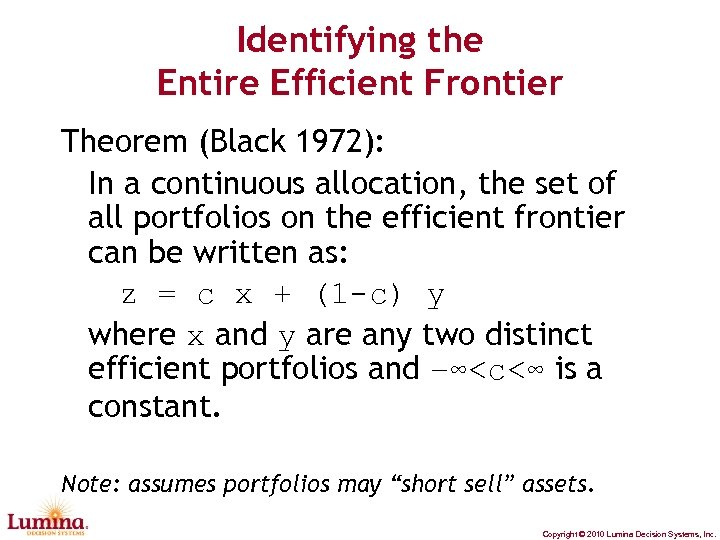Identifying the Entire Efficient Frontier Theorem (Black 1972): In a continuous allocation, the set of all portfolios on the efficient frontier can be written as: z = c x + (1 -c) y where x and y are any two distinct efficient portfolios and –∞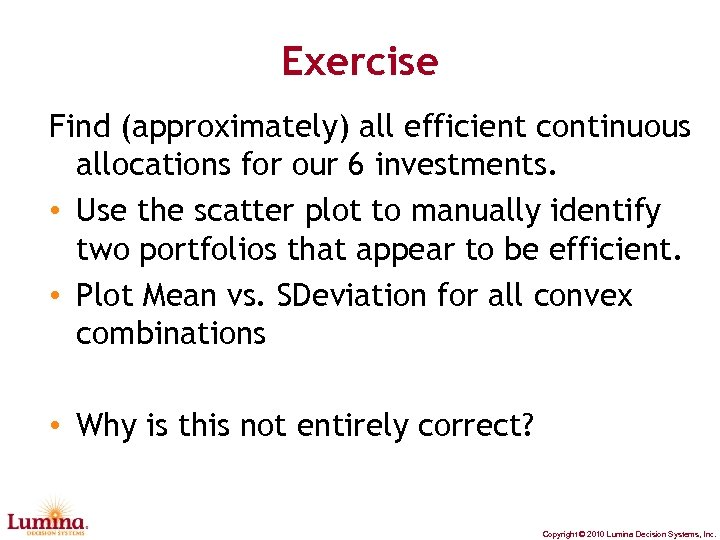Exercise Find (approximately) all efficient continuous allocations for our 6 investments. • Use the scatter plot to manually identify two portfolios that appear to be efficient. • Plot Mean vs. SDeviation for all convex combinations • Why is this not entirely correct? Copyright © 2010 Lumina Decision Systems, Inc.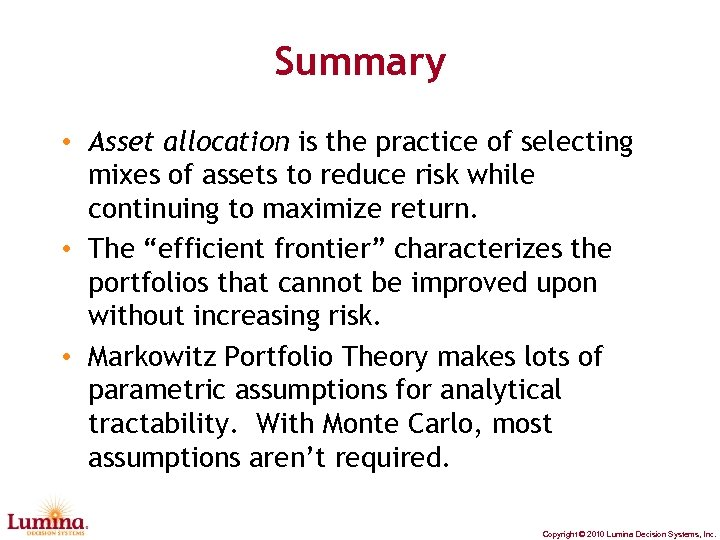Summary • Asset allocation is the practice of selecting mixes of assets to reduce risk while continuing to maximize return. • The “efficient frontier” characterizes the portfolios that cannot be improved upon without increasing risk. • Markowitz Portfolio Theory makes lots of parametric assumptions for analytical tractability. With Monte Carlo, most assumptions aren’t required. Copyright © 2010 Lumina Decision Systems, Inc.